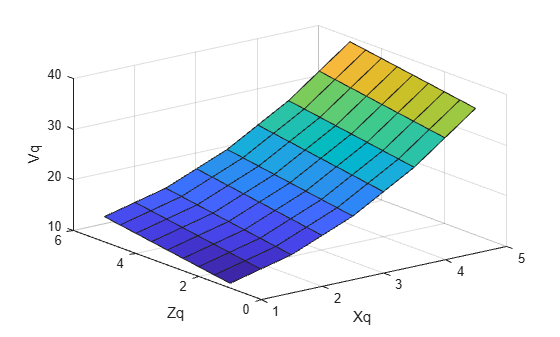# Interpolation of 2-D Selections in 3-D Grids

This example shows how to reduce the dimensionality of the grid plane arrays in 3-D to solve a 2-D interpolation problem.

In some application areas, it might be necessary to interpolate a lower dimensional plane of a grid; for example, interpolating a plane of a 3-D grid. When you extract the grid plane from the 3-D grid, the resulting arrays might be in 3-D format. You can use the `squeeze` function to reduce the dimensionality of the grid plane arrays to solve the problem in 2-D.

Create a 3-D sample grid and corresponding values.

```[X,Y,Z] = ndgrid(1:5); V = X.^2 + Y.^2 +Z;```

Select a 2-D sample from the grid. In this case, the third column of samples.

```x = X(:,3,:); z = Z(:,3,:); v = V(:,3,:);```

The 2-D plane occurs at `Y=3`, so the `Y` dimension has been fixed. `x`, `z`, and `v` are 5-by-1-by-5 arrays. You must reduce them to 2-D arrays before evaluating the interpolant.

Reduce `x`, `z`, and `v` down to 2-D arrays using the `squeeze` function.

```x = squeeze(x); z = squeeze(z); v = squeeze(v);```

Interpolate the 2-D slice over a finer grid of query points.

```[Xq,Zq] = ndgrid(1:0.5:5); Vq = interpn(x,z,v,Xq,Zq);```

Plot the results.

```figure surf(Xq,Zq,Vq); xlabel('Xq'); ylabel('Zq'); zlabel('Vq');```